# 1. Kinematics

## Introduction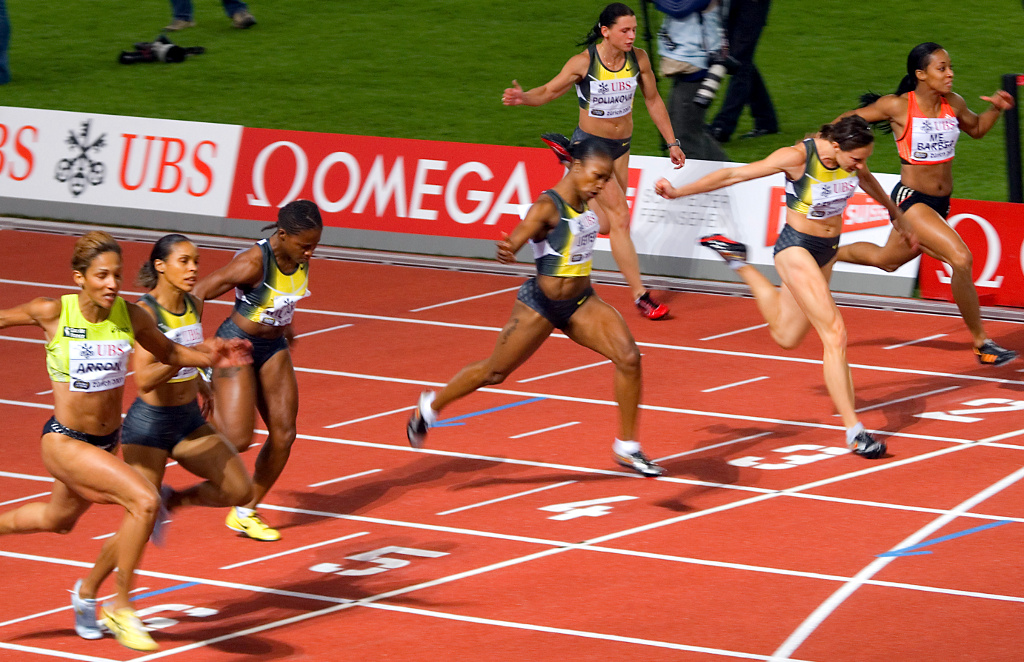Kinematics is the study of motion without considering its causes. In the case of the runners in the photograph, the motion of their legs and arms is oscillatory, while their heads' motion is more similar to rectilinear uniform motion being then easier to describe; it would be enough to follow the horizontal displacement of a point in the head at different instants. To describe the motion of the legs, besides the horizontal displacement, it would also be necessary to measure an angle at different instants.

## 1.1. Rigid bodies motion

An object is in motion if its position is different at different instants; if the position remained the same, the object would be at rest. To determine the position of an object it is necessary to use a reference frame; namely, other objects used as reference. If the position of the object changes with respect to that reference frame, the body has motion relative to that frame. Therefore, motion is a relative property, since a body can be in motion relative to a reference frame and also at rest relative to another reference frame.

The simplest motion that a rigid body can have is a translation without rotation, when all of its parts follow exactly the same trajectory (see Figure 1.1). Hence, in that case it would suffice to describe the motion of a single point in the body in order to follow the motion of the whole rigid body.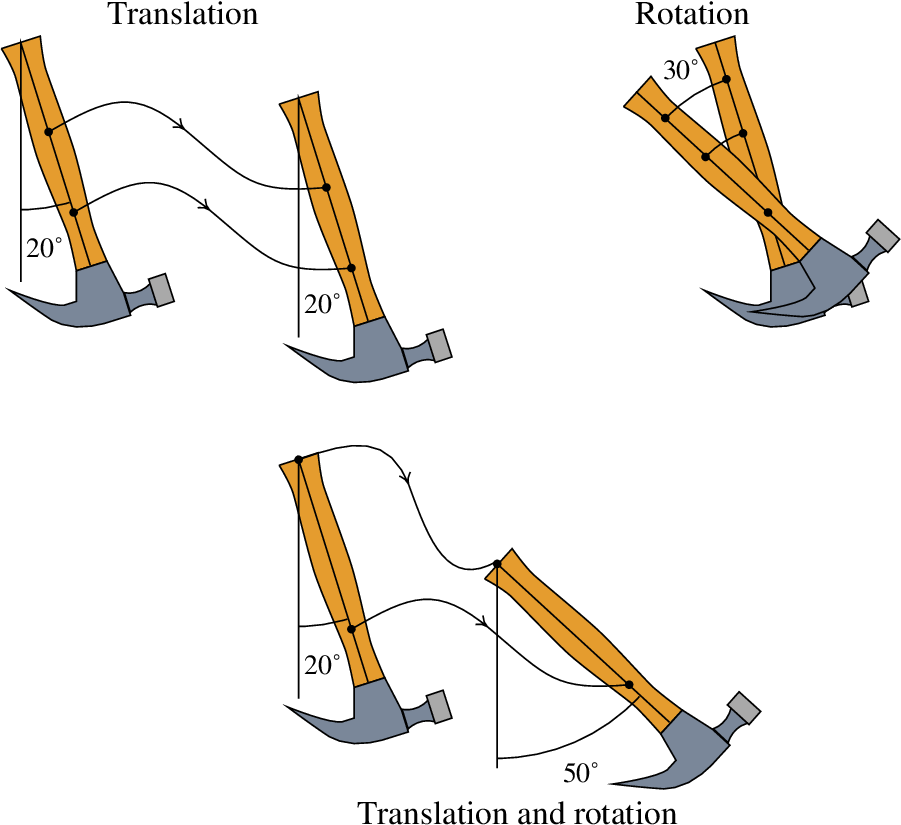Figure 1.1: Translation, rotation around a fixed axis and the combination of those two motions.

In the rotation around a fixed axis, all the points in an axis remain at rest and the other points move. In the second part of Figure 1.1, the hammer rotated around an axis perpendicular to the page. The trajectories of different points are no longer the same, but they are all circular arcs, with the same angle, which only differ in the length of the radius. It would be enough to know how the angle changes with time, in order to describe the motion of any point in the body.

A more complicated motion is obtained from the superposition of a translation and a rotation around a fixed axis (third part in Figure 1.1). In that case, the trajectories of different points are all different curves. However, that complicated motion can be obtained from the trajectory of any point in the body and the rotation of any line segment in the body (not parallel to the rotation axis). All line segments in the body rotate at the same rate and once the position of the reference point and the rotation angle are known at a given instant, the position of all other points in the body can be found at that instant.

There is also a more general type of rotation, around a point, in which the direction of the rotation axis changes with time. In that case the trajectories of all points relative to a reference point will be different curves on the surfaces of spheres centered in that point. A convenient way to describe that kind of motion consists in using two angles to determine the direction of the axis; together with the rotation angle around that axis, a total or three angles are needed in addition to three distances to determine the position of the reference point.

## 1.2. Motion and degrees of freedom

The degrees of freedom of a system are the variables needed to determine its exact position. For example, to determine the position of a fly in a "rectangular" room, its distance to the floor and to two perpendicular walls can be measured, giving rise to a system of perpendicular coordinates (Cartesian or rectangular coordinates), which are usually labeled with the three letters , and (figure 1.2).

That means that the motion of a point in space has 3 degrees of freedom. The trajectory of the point is a curve in space, which can be described by 3 expressions for the 3 Cartesian coordinates , and as functions of time. Since the most general motion of a rigid body can be described by the change of three angles and the motion of a point, that kind of motion has 6 degrees of freedom. Other simpler motions of a rigid body have less degrees of freedom; the rotation around a fixed axis has only one degree of freedom, translation without rotation has 3 degrees of freedom and the superposition of translation and fixed axis rotation has 4 degrees of freedom.

The subject of study in this chapter is the motion of a single point, which will be enough in order to study the translation of rigid bodies and will be the basis to study their general motion.

When a point is restricted to move along a predetermined curve, the motion of that point has only one degree of freedom. For instance, the motion of each wheel of a cart in a roller coaster will follow the curve of the tracks, as long as the cart does not fall off the tracks, so the center of the wheels will follow a known curve. Given the position of that point at an initial instant, to find out the position at a later instant it will be enough to know the distance that the point moved along its trajectory.

When a car moves on a road, one single degree of freedom might be enough to describe its motion (Figure 1.3). If the car breaks down and the driver has to call a towing truck, it will be enough to tell the truck driver the road where the car is and the kilometer along that road. Hence, the motion of cars on a road has one degree of freedom, which is the displacement along the road.Figure 1.3: The translation of a car along a road can be described with only one degree of freedom.

It must be pointed out that the displacement along the road is not measured in a straight line, but along a curve in space; however, since that curve is fixed, only one variable is enough to establish the position at a given instant. In some cases it might become necessary to describe the variation of other degrees of freedom, for instance, the distance from the car to the road's edge. If the car was perfectly rigid and its wheels remained always in contact with the road's surface, the complete description of it s motion would also require an angle. In practice, there are always many degrees of freedom because objects are never perfectly rigid.

If a point is constrained to move on a surface, two coordinates will be enough to determine its position and thus, its motion has two degrees of freedom. A biologist tacking the motion of a fox in a region will only need to measure its latitude and longitude, for instance with a GPS device, in order to know where it is at different instants. Only 2, rather than 3, coordinates are needed because the topography of the terrain is known, as in Figure 1.4, making it possible to determine its exact position just from its latitude and longitude; there is really a third coordinate, the height over the terrain, but since its variation might be insignificant when compared with the variations in the latitude and longitude, it will probably be irrelevant to the study.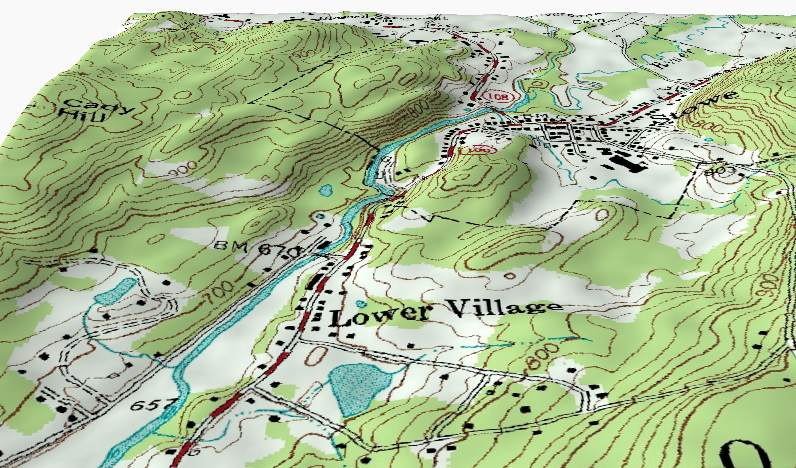Figure 1.4: The translation on the surface of a terrain is a motion with two degrees of freedom.

Therefore, the translation of the fox has two degrees of freedom, because two variables are enough to determine its position. The latitude and longitude are not really distances but angles with their vertices at the center of the Earth, but they are degrees of freedom because can have different values at different instants.

Going back to the example of the fly in a room, which was regarded as having only three degrees of freedom , e , the fly can also change its orientation in space. To determine its orientation in any instant 2 angles might be used and a third angle is needed to determine its rotation along that axis. And since the fly can also stretch or bend its body and open or close its wings, from a mechanical point of view it has several degrees of freedom. If it was modeled by three rigid bodies: two wings and the block formed by its abdomen, thorax and head, 6 degrees of freedom would be needed to describe the motion of that block; each wing will add other 3 degrees of freedom — the three rotation angles with respect to the point where the wing joins the thorax — leading to a total of 12 degrees of freedom.

## 1.3. Displacement and velocity

This chapter deals only with motions with a single degree of freedom, when the trajectory is a known curve. To determine the position along the trajectory, a variable is used, which is measured from a point chosen as the origin (where ); the points on one side of that origin are given positive values of and the points on the other side are given negative values of .

The position is a function of time, because at each instant the point can be in one position only and it is a continuous function because the point cannot move from one position to another without first passing through all the positions in between. At an instant later than ( ), the point will be in another position . The increment of its position during that time interval is called displacement and it is given by

(1.1)

The average velocity, during that time interval is defined as the displacement divided by the time interval

(1.2)

The displacement and the average velocity can be positive or negative, but both have the same sign. If they are positive, it means that the motion is in the positive direction (in which increases) and if they are negative, the motion is in the negative direction. The absolute value of is the average speed of the object. Velocity and speed are measured in units of distance over time; for instance, meters per second, m/s, or kilometers per hour, km/h.

### Example 1.1

A car driver registered his position in a highway at several instants, obtaining the data shown in the table.

 time (h) 0 0.5 1 1.5 2 distance (km) 0 60 90 100 140

Compute the average velocity in each half-hour interval and make the plots of position vs time and average velocity vs time.

Solution. If , , ..., are the five instants registered in the table, the average velocities in the four intervals are the following

The plot of position vs time can be made with Maxima (see appendix A). It is convenient to save the time and position data into a list that will then be used to make the plot with function plot2d.

(%i1) s_t: [[0,0], [0.5,60], [1,90], [1.5,100], [2,140]]\$
(%i2) plot2d ([discrete, s_t], [style, points], [xlabel, "t (h)"], [ylabel, "s (km)"])\$

The result is shown in Figure 1.5. Since should be a continuous function, its plot should be a curve that goes through the points shown in the figure; however, the information given is not enough to know that curve.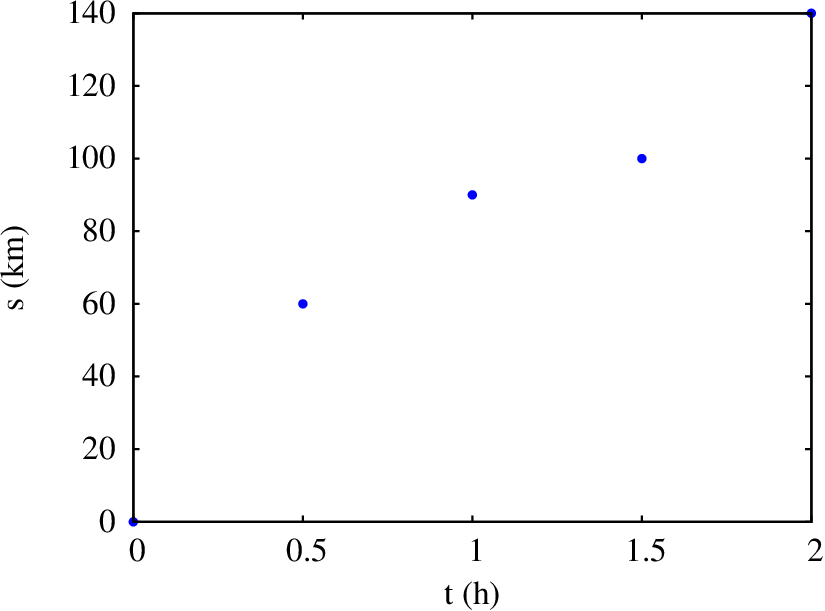Figure 1.5: Plot of position on the trajectory at some instants.

To plot the average velocity with respect to time, it should be kept in mind that each average velocity was computed for a time interval and, hence, each value obtained should be associated to all the instants in that interval. The following two commands create a list with the average velocities with respect to time that is then used to make the plot using plot2d. It is not necessary to use option style, because by default the points given will be connected by line segments.

(%i3) v_t: [[0,120],[0.5,120],[0.5,60],[1,60],[1,20],[1.5,20], [1.5,80],[2,80]]\$
(%i4) plot2d ([discrete, v_t], [x,0,2], [y,0,150], [xlabel,"t (h)"],[ylabel,"v (km/h)"])\$

Figure 1.6 shows the result.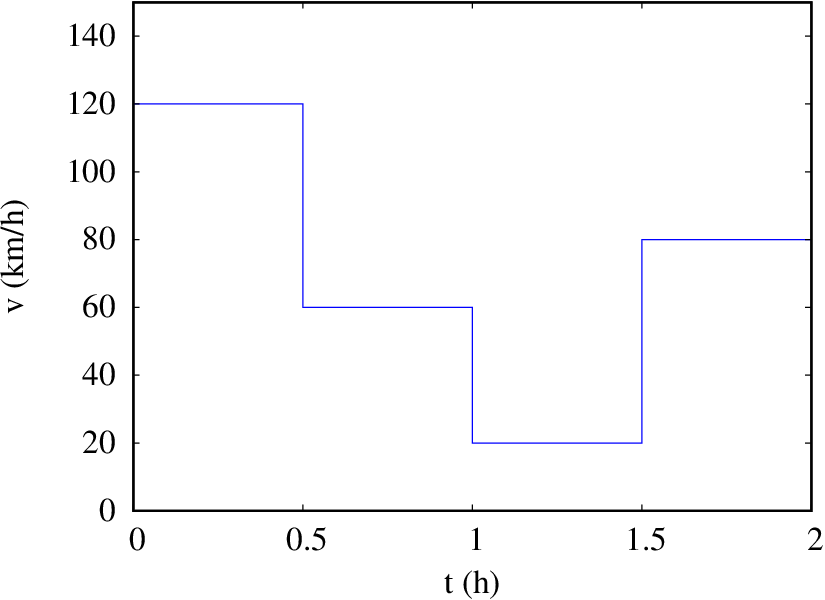Figure 1.6: Plot of the average velocity in several time intervals.

The plot 1.6 does not provide precise information about the true motion of the car. For instance, in the second time interval from 0.5 to 1 hour, instead of traveling at a constant velocity of 60 km/h, as the plot suggests, the driver might have kept the 120 km/h velocity of the first half hour during 15 minutes and then stop for the remaining 15 minutes in that interval; thus, during the first 15 minutes of that second interval the car would have traveled 30 km, reaching the  km position registered in the table.

The position is calculated from the average velocity by combining equations 1.1 and 1.2

(1.3)

The second term in this last equation is the displacement during the time interval . Splitting that interval into subintervals, this last equation would become

(1.4)

Where is the average velocity in the subinterval. Therefore, the average velocity of 60 km/h in the interval  h, leads to a displacement  km, which is the same that would be obtained with an average velocity  km/h during  h, followed by a second average velocity , during  h.

But the velocity cannot change instantly from 120 km/h to 0 without passing through all the values between 120 and 0. In other words, velocity is also a continuous function of time, , as it is the case for position . To get a better glimpse of the two functions and , the time interval can be divided in many more subintervals and the position should be measured in all those subintervals. In the limit when goes to infinity, the sum in equation 1.4 is called an integral and the following notation is used

(1.5)

Within the integral, without a bar over it stands for the instantaneous velocity , equal to the limit of the average velocity as the time interval approaches zero

(1.6)

This limit is called derivative; the last part of the equation shows a notation used very often for the derivative. In this case it is the derivative of function (position) with respect to (time). In this book another notation will be used more often, in which a derivative with respect to time is indicated by writing a dot on top of the function

(1.7)

Hereafter for the sake of simplicity, the instantaneous velocity at an instant will always be called simply velocity.

In a car, the instantaneous speed (absolute value of the instantaneous velocity) is measured with a good approximation by the speedometer. The speedometer reading will have some error associated with the minimum response time of the device, . A good quality speedometer should have a very small response time; also, the slower the speed changes, the more accurate the reading of the speedometer will be.

## 1.4. Acceleration

Following the same argument that led to the definition of instantaneous velocity, the increment of during a time interval is given by

(1.8)

And the average tangential acceleration is the velocity increment divided by the time interval

(1.9)

These last two equations can be combined to give the velocity at a later instant, in terms of the initial velocity and the average tangential acceleration

(1.10)

Dividing the time interval into subintervals that approach zero, the velocity is then found by integrating the instantaneous tangential acceleration

(1.11)

Where the instantaneous tangential acceleration equals the derivative of the velocity with respect to time and, therefore, it is also equal to the second derivative of the position with respect to time

(1.12)

The acceleration has units of distance over time squared. For instance, meters per second squared, m/s2.

A negative tangential acceleration means that the velocity is decreasing: it might be slowing down, if the motion is in the positive direction, or it might be speeding up if the motion is in the negative direction. Positive tangential acceleration implies that the point is speeding up, if its motion is in the positive direction, or slowing down if it moves in the negative direction. Zero tangential acceleration implies uniform motion (constant velocity)

The term "tangential acceleration", instead of just acceleration, is being used because as it will be explained in chapter 3, this is only one component of the acceleration which also has another component perpendicular to the trajectory. On the other hand, the velocity always follows the direction of the trajectory and for that reason there is no need to call it tangential velocity.

The tangential acceleration is also a function of time, as well as position and velocity. However, unlike position and velocity, the tangential acceleration does not have to be a continuous function. Position and velocity are properties that define the state of an object and that state cannot suffer abrupt changes, while the acceleration is related to external factors that can suddenly appear or disappear. Hence, the derivative of the acceleration will not be defined as another physical quantity.

### Example 1.2

A ship is initially at rest in a channel; at an instant = 0 the engine is turned on during 5 minutes and it is then turned off, letting the ship come to a stop due to the water resistance. The expression for the velocity as a function of time , in SI units, is

Find the expressions for the tangential acceleration and the position in the trajectory as a function of time. Plot the velocity, tangential acceleration and position with respect to time. Compute the distances traveled by the ship while the engine was on and after it was turned off.

Solution. First, notice that the expression given for the velocity is a continuous function as it should be. The tangential acceleration is obtained differentiating the expression for the velocity. In order to make the computation in Maxima, one can start by saving the two expressions given for the velocity into two different variables

(%i5) v1: 12*(1-exp(-3*t/50))\$
(%i6) v2: 12*(1-exp(-18))*exp(18-3*t/50)\$

The derivative is computed using function diff

(%i7) a1: diff (v1, t);
(%o7)
(%i8) a2: diff (v2, t);
(%o8)

Notice that the tangential acceleration is discontinuous in this case. At , the expression a1 approaches , which is a positive number, while a2 approaches , which is negative. There is a discontinuity in the acceleration at  s, because at that instant the engine was suddenly turned off.

To obtain the expression for the position at any instant , equation 1.5 is used, replacing the initial instant by 0 and the final instant + by ; the initial position can be chosen as equal to 0. For less than or equal to 300, the expression that should be integrated is the first expression given for

For greater than 300 the initial time in equation 1.5 can be fixed as 300 and the initial position becomes , given by the expression for , and the second expression given for should be integrated

Those two integrals are computed in Maxima as follows

(%i9) s1: expand(integrate(v1, t, 0, t));
(%o9)
(%i10) s2: subst(t=300, s1) + expand(integrate(v2, t, 300, t));
(%o10)

Therefore, the expression for the position is (where the origin is at the initial position)

The plot of the velocity is obtained with the following command

(%i11) plot2d(if t<300 then v1 else v2,[t,0,400],[ylabel,"v"], [y,0,14]);

The result is shown in Figure 1.7.

The plot of the tangential acceleration is obtained with

(%i12) plot2d(if t<300 then a1 else a2,[t,0,400],[ylabel,"a"]);

The result appears in Figure 1.8.

Finally, the following command produces the plot of the position

(%i13) plot2d(if t<300 then s1 else s2,[t,0,400],[ylabel,"s"]);

The result is shown in Figure 1.9.

The plots provide useful information that is less obvious in the algebraic expressions. The plot of the velocity shows that the ship quickly approaches a maximum velocity of around 12 m/s during the first minute, which remains constant until the moment when the engine is turned off. From that moment on, the velocity decreases quickly and at  s (6 minutes) it is practically zero.

The plot of the tangential acceleration shows that when the motor is turned on, it produces an acceleration of approximately 0.7 m/s2 that quickly decreases. When the motor is switched off, the tangential acceleration suddenly becomes approximately −0.7 m/s2 and the way its absolute value decreases with time appears to be identical to the way the initial positive acceleration decreased, the reason being that both acceleration decreases are due to the water resistance. That similarity of the plot of the acceleration when the motor is turned on and when it is turned off, implies that the area between the curve for and the axis is the same in both cases; therefore, the integral of while the motor was on and off are both equal, but with opposite signs and that implies that the speed that the ship gains while the motor is on equals the speed that it looses until it stops, as it should be.

In practice, the expression given for the velocity cannot be completely true when it leads to small values; for instance, at  s the expression for the velocity gives

(%i14) float (subst (t=400, v2));
(%o14)     0.02975

almost 3 centimeters per second. There are other factors, such as water currents, wind and waves, that will introduce velocity variations greater than that value. The expression given is a mathematical model that is only valid when all those other factors can be neglected.

The discontinuity on the plot of the tangential acceleration at  s appears as a vertical segment because Maxima's command plot2d does not discover the discontinuity at that point, but regards the two parts in the plot as a single continuous function. The plot of the position shows a linear increase in almost all of the first 5 minutes. The distance traveled while the motor was turned on equals the displacement from to ; since the initial position is , that displacement is

According to the theoretical model, the ship would take an infinite time to stop; in practice, it stops after about 6 minutes, as it was discussed above. As such, the distance traveled after the motor was turned off would be . The limit of as approaches infinity is calculated in Maxima as follows

(%i15) limit (s2, t, inf);
(%o15)     3600

It is then concluded that the ship travels 200 m since the engine is turned off until stops.

## 1.5. Uniform and uniformly accelerated motion

A motion is called uniform if the velocity remains constant. Since the derivative of a constant is zero, the tangential acceleration is then zero. The result obtained from equation 1.4 will be the same, independently of the number of subintervals, because is the same in all the subintervals (the average velocity equals the velocity in this case) and that result is

(1.13)

where is the position at instant , is the initial position at e the velocity, which is the same at any time.

A uniformly accelerated motion is one which has a constant tangential acceleration. In that case, the average tangential acceleration in equation 1.10 can be replaced by the constant value of the tangential acceleration leading to

(1.14)

where is the velocity in . The expression for the position is obtained by substituting this equation into equation 1.5

(1.15)

The time can be eliminated from equations 1.14 and 1.15, leading to an expression that relates velocity and position

(1.16)

It should be remembered that equations 1.14, 1.15 and 1.16 are only valid in the case of a constant tangential acceleration. When the tangential acceleration is not constant, in order to obtain an expression for the velocity from equation 1.11 it would be necessary to know as a function of . And the expression obtained for could then be integrated in equation 1.5, leading to an expression for which will not have the same quadratic form 1.15. If the expression for as a function of time is not known, in some cases the method explained in the next section can be used.

## 1.6. Kinematic equations

The differential equations 1.7 and 1.12 define the relation among the kinematic variables ( , , ) and the time . If the expression for one of those variables as a function of time is known, the expressions for the other two variables can be found just by differentiation or integration, as it was done in the example 1.2.

When an expression for as a function of is known, the derivative of with respect to can be obtained by the rule for differentiation of composed functions

(1.17)

This is another relation among three of the four kinematic variables. To summarize, the four equations that relate three of the four kinematic variables , , and are (notice that the equations with dots include the variable )

(1.18)

Any of those equations can be solved when there is a known relation among some of the three variables in the equation. For instance, let us consider the first equation, ; if an expression for the position in terms of time is known, namely , the equation becomes

(1.19)

which implies that the expression for in terms of is the derivative of the function given. Notice that if the function depended on other variables, for instance or , the velocity would not be just the derivative of , since the derivative must be computed using the the chain rule:

(1.20)

In the first case, an expression for the acceleration in terms of the velocity is obtained and in the second case one only finds a relation involving the acceleration, the velocity and the time. In cases when an expression for in terms of is known, , as in the example 1.2, the equation becomes

(1.21)

which is equivalent to (as in equation 1.5):

(1.22)

In more complicated cases, for instance if the relation known is of the form , it is obtained an ordinary differential equation, namely, an equation with two variables and the derivative of one of them with respect to the other. A similar analysis can be made with the other 3 equations.

Some differential equations can be solved analytically using various known methods, as shown in the next example, but in some other cases the solution must be obtained numerically, which will be discussed in chapter 7.

### Example 1.3

When an arrow is thrown with a bow (see the figure), while the arrow is in contact with the string its tangential acceleration decreases linearly with respect to its position , from a maximum value of 4500 m/s2, when the back of the arrow is in the initial position A, down to zero when it reaches the point B where the bow recovers its normal shape. The distance between points A and B is 600 mm. Determine the velocity of the arrow as it leaves the bow in B.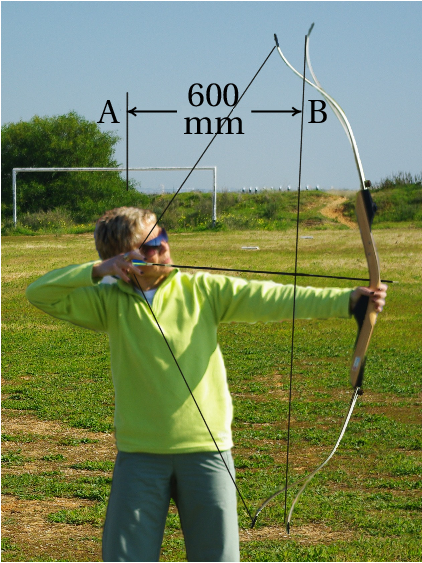Solution: Using point A as the origin for the position of the arrow and SI units, the expression for the tangential acceleration in the interval 0 ≤ ≤ 0.6 m is the equation of the straight line that goes through points ( , ) = (0, 4500) and ( , ) = (0.6, 0), namely

This expression can be substituted in the equation

leading to an ordinary differential equation

Moreover, this kind of equation is called separable, because the two variables can be separated in the two sides of the equation in the following way

If = 0 is chosen as the instant when the arrow is released at point A, then the initial condition necessary to solve this equation is that = 0 when = 0. Integrating on each side of the equation, from those initial values up to the values in point B, one has

Notice that the limits in both integrals must be consistent; namely, each limit in the integral with respect to is the value of in a point and the corresponding limit in the integral with respect to on the other side of the equation is the value of at that same point. Solving the two integrals, the value of at point B is obtained

### 1.6.1. Projection along a straight axis

In some cases it is more convenient to study the motion of a point along a curve by studying the motion of its projection along a straight line which is considered, for instance, the axis.

In an interval , the displacement of the projection along the axis will be . Therefore, the derivative of the projection with respect to time is the projection of the velocity along the axis, . And the derivative is the projection of the acceleration along the axis, . Notice that = 0 does not imply zero velocity, because it might happen that the trajectory is perpendicular to the axis at some point, making the projection of the velocity equal to zero in that point. Also, is the projection of the total acceleration and not only its tangential component; for instance, can be constant, implying equal to zero, but if the trajectory is curved, the projection of can be different at different points, implying that is not equal to zero.

The equations that relate , , and are similar to the equations 1.18 for the motion along the trajectory

(1.23)

In the particular case of rectilinear motion, when the trajectory is a straight line, and if the axis is chosen parallel to the trajectory, then is equal to the position in the trajectory, is the velocity and is the total acceleration, rather than just its tangential part. The axis can be called , or anything else instead of .

### 1.6.2. Acceleration of gravity

As Galileo Galilei explained in his book of 1638, "Dialogues Concerning Two New Sciences", the motion of a projectile thrown in the air can be conveniently decomposed into two motions: the motion of the its projection along the horizontal plane, and the motion of its projection along a vertical axis. Figure 1.10 is the same original figure 108 from Galileo's book of an object that was thrown from point a on a horizontal surface, leaving that surface at point b.

Galileo also discovered that when the air resistance can be neglected, which is true when the object has a compact shape and its trajectory in the air is not too long, the motion of the horizontal projection is rectilinear and uniform, namely, in equal intervals of time the object moves from a to b, then from b to c, from c to d and from d to e. In the vertical direction, the distance the projectile falls in equal intervals of time increases quadratically; namely, during the first interval it falls the distance , during the second interval it has already fallen the distance , which is four times bigger than and during the third interval the distance , which is nine times bigger than . Thus, the vertical projection of the acceleration is constant.

When the air resistance can be neglected, all objects, independently of their size and masses, are accelerated downwards with the same acceleration , called gravity. The vertical projection of the motion is a uniformly accelerated motion, while the horizontal projection is a uniform motion.

The value of the acceleration of gravity is slightly different in different places on Earth, but it is approximately 9.8 m/s2.

If the vertical is represented by the axis, pointing upward, then the vertical projection of the acceleration is  m/s2 and its horizontal projection is = 0.

### Example 1.4

A stone is thrown from a bridge that is 5 m above the surface of a river, with an initial velocity of 15 m/s at an angle of 36.9° over the horizontal. Find the velocity that the stone will have when it hits the surface of the river and the maximum height of its trajectory, measured from the surface of the river (assume that the air resistance can be neglected).

Solution. The horizontal projection of the initial velocity is m/s and the vertical projection is m/s. It is convenient to choose the axis horizontal and in the direction of the horizontal projection of the initial velocity and the axis vertical and in the direction of the vertical projection of the initial velocity. The origin might be chosen at the exact point where the stone is thrown or, as it will be done here, directly under that point and at the surface of the river. In that system of coordinates, the initial position is then and (in SI units) and the projections of the acceleration are and .

The two motions along the 2 axes are then analyzed independently. Since the motion along the axis is uniformly accelerated, equations 1.14, 1.15 and 1.16 could be used. However, it will be shown here how to solve the problem using the method of separation of variables, which can be applied to more general cases.

The constant value of can be replaced into the second and the fourth equations in 1.23 (with instead of ), leading to two first-order ordinary differential equations:

To obtain the velocity of the stone when if reaches the surface of the river, the second of those equations should be solved by separating the two variables and on both sides of the equation

Next, the left-hand side of the equation is integrated from the initial height until the final height and the right-hand side is integrated from the initial velocity until and unknown final velocity

Computing these two integrals (in Maxima that is done with integrate (9.8, y, 5, 0) and integrate (vy, vy, 9, vf)), the result is

(the second solution, , indicates the velocity that the stone should have had at the surface of the river, in order to pass through the bridge with a vertical component of the velocity equal to 9 m/s and pointing upwards).

Hence, the vertical component of the stone's velocity when it reaches the river's surface is = −13.38 m/s; since the horizontal component of the velocity remains constant, equal to 12.0 m/s, the velocity of the stone when it reaches the river surface is

At the point of maximum height in the stone's trajectory, the vertical projection of the velocity is equal to zero. The same two integrals calculated above can be calculated again, leaving the final value of as an unknown (maximum height ) and replacing the final limit of by 0

solving these integrals, the maximum height is found

The method of separation of variables can only be used to solve some ordinary differential equations, when the two variables can be separated. There are various other analytical methods that can be used for other types of equations, but not all equations can be solved analytically. The approach adopted in the next chapters of this book will be to use numerical methods to solve any differential equation that cannot be solved by separation of variables.

## Questions

1. The tangential acceleration of an object is (SI units), where is the time. If at the initial instant = 0 the velocity of the object is 4 m/s, what will be its velocity 3 seconds later?
1. 22 m/s
2. 18 m/s
3. 40 m/s
4. 36 m/s
5. 4 m/s
2. In which of the following cases there is no doubt that the object is slowing down?
1. = 3 m/s, = 5 m/s2
2. = −3 m/s, = 5 m/s2
3. = 3 m/s, = 5 m/s2
4. = −3 m/s, = 5 m/s2
5. = −3 m/s, = −5 m/s2
3. The expression for the velocity of a particle as a function of the position on the trajectory is . What is the correct expression for its tangential acceleration?
4. The figure shows the plot of the velocity of a body as a function of time. Find the distance traveled from to  s.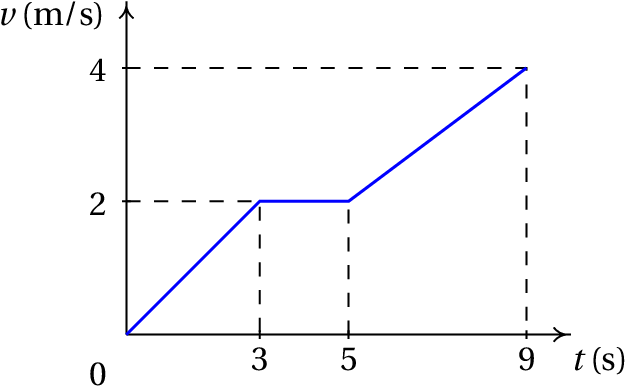1. 1 m
2. 12 m
3. 7 m
4. 5 m
5. 19 m
5. In a plot of the velocity as a function of position on the trajectory, what does the slope of the curve represent?
1. The tangential acceleration.
2. The velocity.
3. The tangential acceleration divided by the velocity.
4. The product of the velocity and the tangential acceleration.
5. The velocity divided by the tangential acceleration.

## Problems

1. The position of an object in its trajectory is given by the expression (SI units). Determine the time, position and tangential acceleration at all the instants when the object stops (its velocity is = 0).
2. The acceleration of object that moves along the axis is = −4 m/s2. If at = 0, the velocity is = +24 m/s and the object is at the position = 0, find its velocity and position at = 8 s and the total distance traveled by the object from = 0 to = 8 s.
3. At = 0 an object is at rest in the position = 5 cm in a path. From that moment, the object starts to move in the positive direction of the path, stopping again at . The expression for the tangential acceleration between and is , where time is measured in seconds and the acceleration in cm/s2. Find: (a) The instant when the object stops. (b) The position along the path at that instant.
4. The expression for the tangential acceleration of a particle is , where is a positive constant. The particle is initially at rest at = 800 mm and it starts moving passing by = 500 mm with a velocity −6 m/s. Find: (a) The value of the constant . (b) The velocity of the particle at = 250 mm.
5. The acceleration of an object that oscillates on the axis is , where is a positive constant. Find:
1. The value that must have so that = 15 m/s at = 0 and = 0 at = 3 m.
2. The velocity of the object at = 2 m.
6. The tangential acceleration of an object is (SI units), where is the position on the trajectory and is a constant. Knowing that the object crosses the origin with velocity = 17 m/s, find the velocity at = 4 m for each of the following values of the constant: (a) = 0, (b) = 0.015, (c) = −0.015.
7. The square of the velocity of an object decreases linearly as a function of its position on the trajectory, as shown in the figure. Compute the distance traveled by the object during the last two seconds before it reaches point B.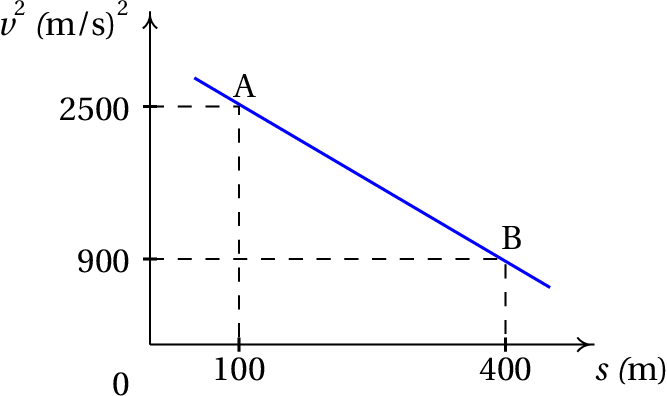8. The tangential acceleration of an object is = −0.4 v, where is measured in mm/s2 and in mm/s. Knowing that at = 0 the velocity is 30 mm/s, find:
1. The distance traveled by the object from = 0 until it stops.
2. The time it took the object to travel that distance.
3. The time when the velocity of the object is 1 percent of the initial velocity at = 0.
9. The position of a particle that moves along the axis is given approximately by the expression (SI units).
1. Find the expressions for the velocity and the acceleration as a function of time.
2. Find the values of the time, position and acceleration in all the instants when the particle is as rest ( = 0).
3. Make the plots of position, velocity and acceleration in the interval 0 ≤ ≤ 20.
10. A projectile is thrown from the top of a a building, 7 m above the ground, with a speed of 15 m/s in a direction 56.3° above the horizontal, as shown in the figure. Assuming that the air resistance can be neglected, find:
1. The time of flight, namely, the time interval since the projectile is thrown until it hits the ground.
2. The horizontal range, namely, the distance in the figure.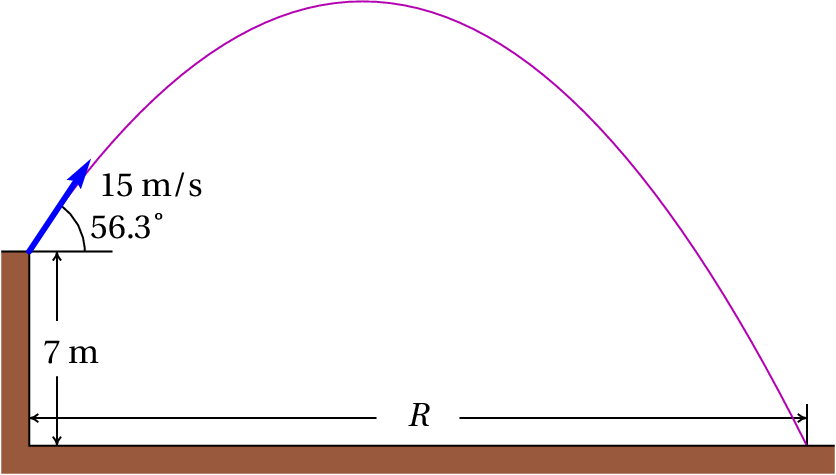11. A marble is thrown over the horizontal surface on the top of some stairs, and it leaves the top of the stairs with horizontal speed of 3 m/s. Each step goes down 18 cm and is 30 cm wide. On which step will the marble land?
12. The expression for the tangential acceleration of an object falling freely through the air is, including the air resistance, , where and are constants. If the object starts falling from rest at = 0,
1. Prove that the velocity at any later time is
2. Find the expression for the velocity of the object after it has fallen a distance .
3. Why is it that the velocity is called terminal velocity?

Questions: 1. A. 2. B. 3. A. 4. C. 5. C.

Problems

1. = 0, = 10 m, = −12 m/s2 and = 2 s, = 2 m, = 12 m/s2.
2. Velocity −8 m/s, position = 64 m and distance traveled equal to 80 m.
3. (a) 3 s (b) 25.25 cm.
4. (a) 24 m3/s2 (b) 11.49 m/s.
5. (a) 25 s−2 (b) ±11.18 m/s (the object oscillates).
6. (a) ±15 m/s, because the object oscillates. (b) ±14.74 m/s, because the object oscillates. (c) 15.25 m/s, only positive because the object moves always in the positive direction. (To find out whether the object oscillates or not, one can find the expression for as a function of and look at its plot).
7. 65.33 m
8. (a) 75 mm (b) infinite (c) 11.51 s.
1. ,
2. At  s,   m,   m/s2.  Em  s,   m,   m/s2
3. The plots are the following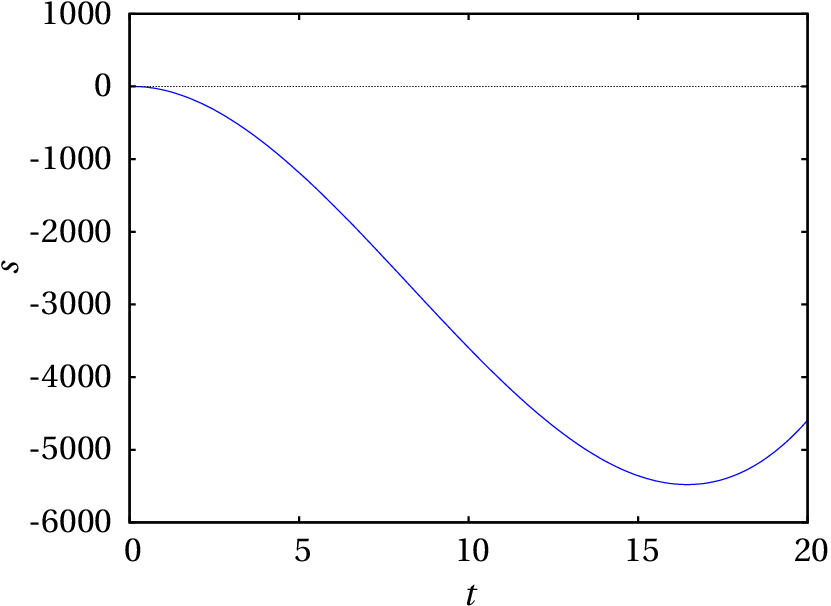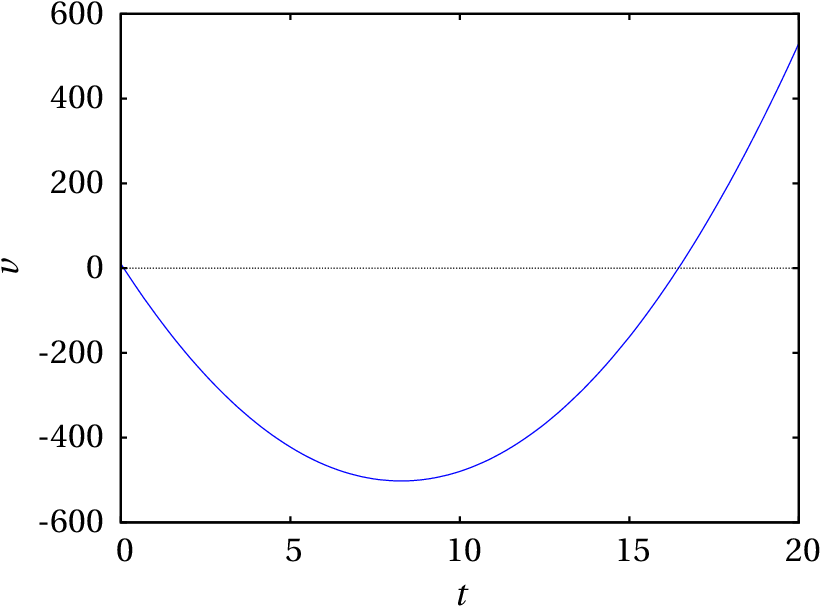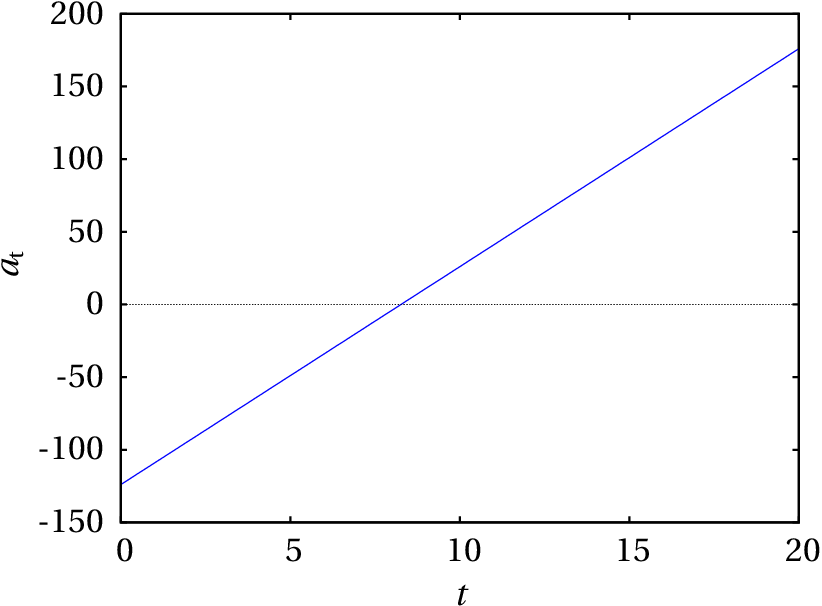9. (a) 3.02 s. (b) 25.1 m.
10. On the fourth step.
11. (b)
(c) Because after a long time approaches:
Correct

The increase of the velocity during the 3 seconds equals the integral of , with respect to time, which should then be added to the initial velocity.

(click to continue)

Wrong

18 m/s is just the increase of the velocity during the 3 seconds. That increase must be added to the initial velocity.

(click to continue)

Wrong

The increase of the velocity during the 3 seconds equals the integral of , with respect to time, which should then be added to the initial velocity.

(click to continue)

Wrong

The increase of the velocity during the 3 seconds is not equal to times , but the integral of , with respect to time, from = 0 to = 3.

(click to continue)

Wrong

the velocity cannot remain constant because there is tangential acceleration, always positive, which implies an increase in the velocity.

(click to continue)

Wrong

Positive tangential acceleration implies increase of the velocity and since the velocity is positive it is then getting bigger and the object is speeding up.

(click to continue)

Correct

Positive tangential acceleration implies increase of the velocity and since the velocity is negative its absolute value is then getting smaller, so the object is slowing down.

(click to continue)

Wrong

It can be concluded that the absolute value of is getting bigger, but that doesn't give any information about the velocity, because it might have other components, such as , that might be decreasing.

(click to continue)

Wrong

It can be concluded that the absolute value of is getting smaller, but that doesn't give any information about the velocity, because it might have other components, such as , that might be increasing.

(click to continue)

Wrong

It can be concluded that the absolute value of is getting bigger, but that doesn't give any information about the velocity, because it might have other components, such as , that might be decreasing.

(click to continue)

Correct

The derivative of with respect to is obtained by multiplying its derivative with respect to , times itself (derivative of with respect to ).

(click to continue)

Wrong

The acceleration is the derivative of with respect to , which is not equal to the derivative with respect to .

(click to continue)

Wrong

The acceleration cannot be obtained dividing by . The equation is only valid when is constant, which is not the case here.

(click to continue)

Wrong

If you obtained this result dividing by , that is not the correct way to compute the tangential acceleration. If you found it as the derivative of the velocity, you made an error in the differentiation.

(click to continue)

Wrong

If you obtained this result from the equation , that equation is only valid when the acceleration is constant (not true in this case) and the initial velocity is zero at = 0 (that part is correct in this case).

(click to continue)

Wrong

The distance traveled is the integral of , from = 0 to = 5, which in the plot corresponds to the area of the triangle from = 0 to = 3, plus the area of the square from = 3 to = 5.

(click to continue)

Wrong

The distance traveled is the integral of , from = 0 to = 5, which in the plot corresponds to the area of the triangle from = 0 to = 3, plus the area of the square from = 3 to = 5.

(click to continue)

Correct

The distance traveled is the integral of , from = 0 to = 5, which in the plot corresponds to the area of the triangle from = 0 to = 3, plus the area of the square from = 3 to = 5.

(click to continue)

Wrong

The distance traveled is the integral of , from = 0 to = 5, which in the plot corresponds to the area of the triangle from = 0 to = 3, plus the area of the square from = 3 to = 5.

(click to continue)

Wrong

The distance traveled is the integral of , from = 0 to = 5, which in the plot corresponds to the area of the triangle from = 0 to = 3, plus the area of the square from = 3 to = 5.

(click to continue)

Wrong

The slope of the plot represents the derivative , which is not necessarily equal to .

(click to continue)

Wrong

The slope of the plot represents the derivative , while the velocity is the derivative

(click to continue)

Correct

The slope of the plot represents the derivative , equal to

(click to continue)

Wrong

The slope of the plot represents the derivative , with units of 1 over time, while has units of distance squared over time cubed.

(click to continue)

Wrong

The slope of the plot represents the derivative , with units of 1 over time, while has units of time.

(click to continue)